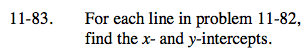Home > AC > Chapter 11 > Lesson 11.2.1 > Problem11-83

11-83.

For each line in problem 11-82, find the x- and y-intercepts. Homework Help ✎Use the equations you found in problem 11-82 to help you find the intercepts.

To find the x-intercepts, substitute 0 for y in the equation. To find the y-intercepts,
substitute 0 for x in the equation or simply read the intercept right off the equations.